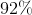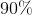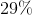# Algebra 1 : How to find percentage equivalent to a decimal

## Example Questions

### Example Question #2951 : Algebra 1

Find the percentage equivalent for the following decimal: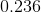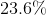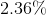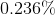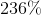Explanation:

In order to find the percentage equivalent for a decimal, all we have to do is multiply our decimal by 100. We can also move the decimal point to the right two spaces.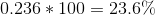### Example Question #2952 : Algebra 1

Find the percentage equivalent of the following decimal: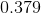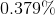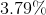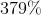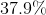Explanation:

In order to find the percentage equivalent for a decimal, all we have to do is multiply our decimal by 100. We can also move the decimal point to the right two spaces.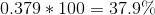### Example Question #23 : Decimals And Percentage

What is the percentage equivalent of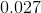?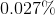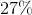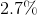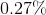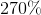Explanation:

To find the percentage equivalent of a decimal, we multiply our given decimal value by one-hundred: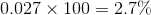### Example Question #21 : How To Find Percentage Equivalent To A Decimal

If you havedollars yet to pay on a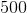dollar loan, what percentage of your loan do you still owe?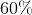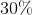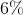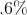Explanation:

To solve this problem, divide the amount owed by the total amount.  This will yield you a decimal.  Then, to find the percentage equivalent of a decimal, simply move the decimal (if there is none, remember that there is always an implied decimal after the one's digit) to the right twice.  The best answer is: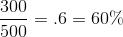### Example Question #25 : Decimals And Percentage

What is the percentage equivalent of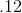?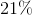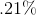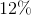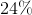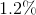Explanation:

To find the percentage equivalent of a decimal, simply move the decimal (if there is none, remember that there is always an implied decimal after the one's digit) to the right twice.  The best answer is:### Example Question #26 : Decimals And Percentage

Which of the following is the percent equivalent of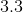?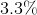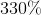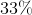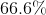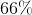Explanation:

To find the percentage equivalent of a decimal, simply move the decimal (if there is none, remember that there is always an implied decimal after the one's digit) to the right twice.  The best answer is:### Example Question #22 : How To Find Percentage Equivalent To A Decimal

If you havedollars yet to pay on adollar loan, what percentage of your loan do you still owe?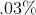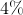Explanation:

To solve this problem, divide the amount owed by the total amount.  This will yield you a decimal.  Then, to find the percentage equivalent of a decimal, simply move the decimal (if there is none, remember that there is always an implied decimal after the one's digit) to the right twice.  The best answer is: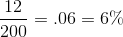### Example Question #23 : Decimals And Percentage

Which of the following is the percent equivalent of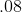?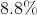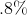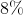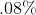Explanation:

To find the percentage equivalent of a decimal, simply move the decimal (if there is none, remember that there is always an implied decimal after the one's digit) to the right twice.  The best answer is:### Example Question #29 : Decimals And Percentage

Which of the following is the percent equivalent of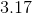?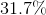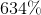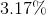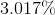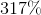Explanation:

To find the percentage equivalent of a decimal, simply move the decimal (if there is none, remember that there is always an implied decimal after the one's digit) to the right twice.  The best answer is:### Example Question #23 : How To Find Percentage Equivalent To A Decimal

If you havedollars yet to pay on adollar loan, what percentage of your loan have you already paid?  Round your answer to the nearest whole percent.